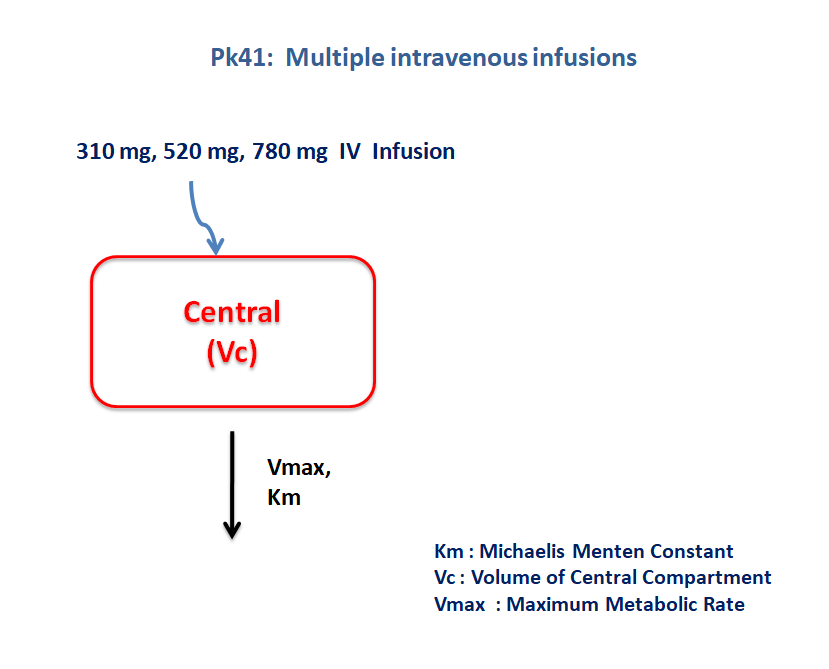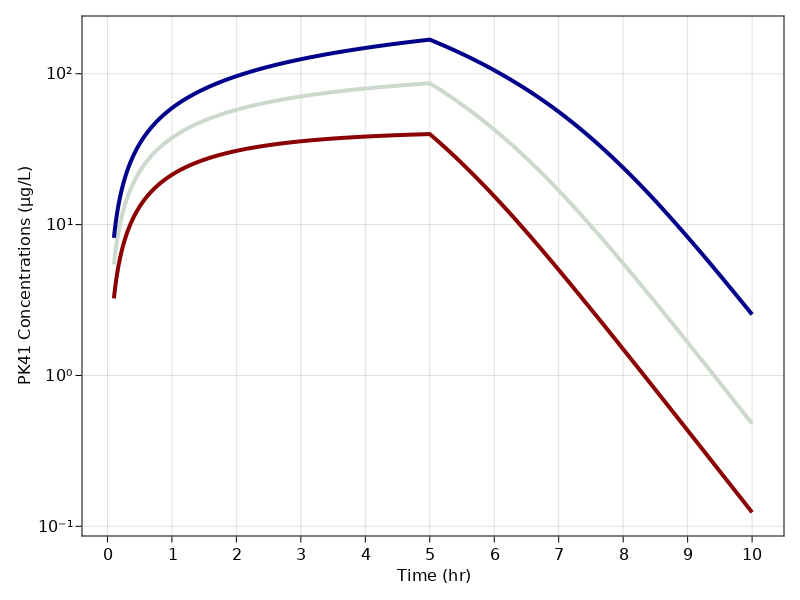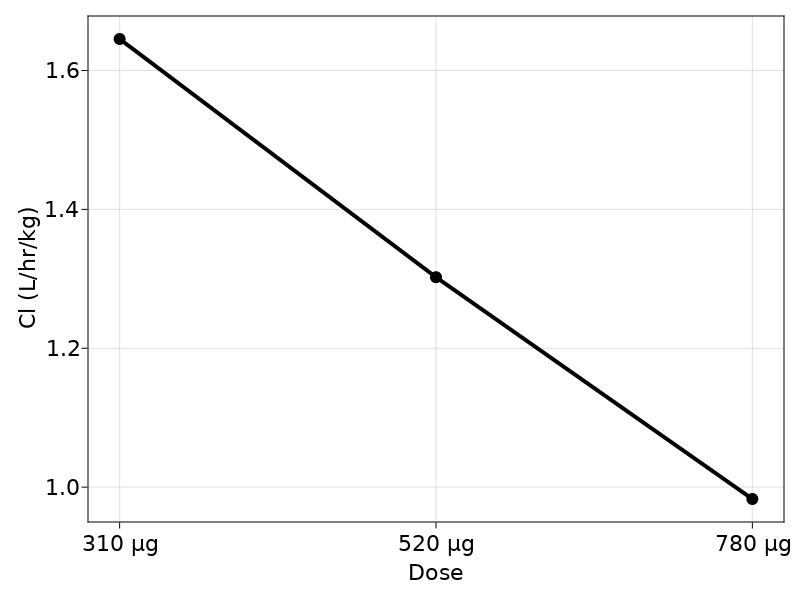# Exercise PK41 - Multiple intravenous infusions - NCA vs regression

### Background

• Structural Model - One compartment model with non-linear elimination.

• Route of administration - IV infusion

• Dosage Regimen - 310 μg, 520 μg, 780 μg

• Number of Subjects - 3### Learning Outcome

This is a one compartment model with capacity limited elimination. Concentration time profile was obtained for three subjects administered with three different dosage regimens.

### Objectives

In this tutorial, you will learn how to build one compartment model with non-linear elimination.

### Libraries

Call the "necessary" libraries to get start.

using Random
using Pumas
using PumasUtilities
using CairoMakie
using AlgebraOfGraphics
using DataFramesMeta


### Model

The following model describes the parameters and differential equation for a one-compartment model with capacity limited elimination

pk_41        = @model begin
desc     = "One Compartment Model"
timeu    = u"hr"
end

@param begin
"Maximum Metabolic Rate (μg/kg/hr)"
tvvmax   ∈ RealDomain(lower=0)
"Michaelis Menten Constant (μg/kg/L)"
tvkm     ∈ RealDomain(lower=0)
"Volume of Central Compartment (L/kg)"
tvvc     ∈ RealDomain(lower=0)
Ω        ∈ PDiagDomain(3)
"Proportional RUV"
σ²_prop  ∈ RealDomain(lower=0)
end

@random begin
η        ~ MvNormal(Ω)
end

@pre begin
Vmax     = tvvmax * exp(η)
Km       = tvkm * exp(η)
Vc       = tvvc  * exp(η)
end

@dynamics begin
Central' = - (Vmax * (Central/Vc)/(Km + (Central/Vc)))
end

@derived begin
cp       = @. Central/Vc
"""
Observed Concentrations (μg/L)
"""
dv       ~ @. Normal(cp, sqrt(cp^2*σ²_prop))
end

@observed begin
conc = Central / Vc
nca     := @nca conc
cl       = NCA.cl(nca)
end
end

PumasModel
Parameters: tvvmax, tvkm, tvvc, Ω, σ²_prop
Random effects: η
Covariates:
Dynamical variables: Central
Derived: cp, dv
Observed: cp, dv, conc, cl


### Parameters

The parameters are as given below. tv represents the typical value for parameters.

• $Vmax$ - Maximum Metabolic Rate (μg/kg/hr)

• $Km$ - Michaelis Menten Constant (μg/kg/L)

• $Vc$ - Volume of Central compartment (L/kg)

param = ( tvvmax  = 180.311,
tvkm    = 79.8382,
tvvc    = 1.80036,
Ω       = Diagonal([0.0,0.0,0.0,0.0,0.0]),
σ²_prop = 0.015)

(tvvmax = 180.311, tvkm = 79.8382, tvvc = 1.80036, Ω = [0.0 0.0 … 0.0 0.0;
0.0 0.0 … 0.0 0.0; … ; 0.0 0.0 … 0.0 0.0; 0.0 0.0 … 0.0 0.0], σ²_prop = 0.0
15)


### Dosage Regimen

• Subject-1 receives a dose of 310 μg given as an IV Infusion over 5 hrs

• Subject-2 receives a dose of 520 μg given as an IV Infusion over 5 hrs

• Subject-3 receives a dose of 780 μg given as an IV Infusion over 5 hrs

dose = [310,520,780]
rate_ind = [62,104,156]
ids   = ["310 μg", "520 μg", "780 μg"]
ev(x)   = DosageRegimen(dose[x], cmt = 1, time = 0, rate = rate_ind[x], route = NCA.IVInfusion)
pop3_sub =  map(i -> Subject(id = ids[i], events = ev(i)), 1:length(ids))

Population
Subjects: 3
Observations:


### Simulation

Simulate the plasma concentration of the drug for both the subjects

Random.seed!(123)
sim_pop3_sub = simobs(pk_41, pop3_sub, param, obstimes = 0.1:0.01:10)


### Visualization

f1, a1, p1 = sim_plot(pk_41, sim_pop3_sub,
observations = :cp,
color = :redsblues,
linewidth = 4,
axis = (
xlabel = "Time (hr)",
ylabel = "PK41 Concentrations (μg/L)",
xticks = 0:1:10, yscale= log10))
f1@chain DataFrame(sim_pop3_sub) begin
dropmissing!(:cl)
unique([:id, :cl])
data(_) *
mapping(:id => nonnumeric => "Dose" , :cl => "Cl (L/hr/kg)") *
visual(ScatterLines, linewidth = 4, markersize = 12)
draw(axis = (;  yticks = 0.8:0.2:1.8), figure = (; fontsize = 22,))
end